﻿ 旋转的双原子–玻色爱因斯坦凝聚体基态数值模拟 Numerical Simulations on Ground States for Rotating Two-Component Bose-Einstein Condensates

Advances in Applied Mathematics
Vol.06 No.09(2017), Article ID:23172,14 pages
10.12677/AAM.2017.69144

Numerical Simulations on Ground States for Rotating Two-Component Bose-Einstein Condensates

Ronghua Liu, Yundan Deng, Chunping Pang, Hanquan Wang

School of Statistics and Mathematics, Yunnan University of Finance and Economics, Kunming Yunnan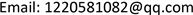Received: Dec. 2nd, 2017; accepted: Dec. 19th, 2017; published: Dec. 26th, 2017ABSTRACT

The ground states of rotating two-component Bose-Einstein condensates (BEC) at extremely low temperature are solutions of time-independent coupled Gross-Pitaevskii equations. To compute the ground states, we propose an efficient numerical method—gradient flows with discrete normalization. In linear cases and under properly chosen initial data, we can prove that the gradient flows converge into the ground state which has the lowest energy. We show that the method is quite efficient and apply the method to study complicated vortex structure in the ground state solutions of rotating two-component BEC at extremely low temperature.

Keywords:Coupled Gross-Pitaevskii Equations, Rotating Two-Component Bose-Einstein Condensates, Gradient FlowsCopyright © 2017 by authors and Hans Publishers Inc.1. 引言

2. 动态相耦合的GP方程组

$i\hslash \frac{\partial {\psi }_{1}}{\partial t}=\left(-\frac{{\hslash }^{2}}{2m}{\nabla }^{2}+{V}_{1}\left(x\right)-\hslash \Omega {L}_{z}+{g}_{11}{|{\psi }_{1}|}^{2}+{g}_{12}{|{\psi }_{2}|}^{2}\right){\psi }_{1}-\hslash {\omega }_{R}{\psi }_{2}$ (2.1)

$i\hslash \frac{\partial {\psi }_{2}}{\partial t}=\left(-\frac{{\hslash }^{2}}{2m}{\nabla }^{2}+{V}_{2}\left(x\right)-\hslash \Omega {L}_{z}+{g}_{21}{|{\psi }_{1}|}^{2}+{g}_{22}{|{\psi }_{2}|}^{2}\right){\psi }_{2}-\hslash {\omega }_{R}{\psi }_{1}$ (2.2)

${V}_{j}\left(x\right)=\frac{m}{2}\left({\omega }_{x,j}^{2}{\left(x-{x}_{j}\right)}^{2}+{\omega }_{y,j}^{2}{\left(y-{y}_{j}\right)}^{2}+{\omega }_{z,j}^{2}{\left(z-{z}_{j}\right)}^{2}\right)$

$N:=N\left({\psi }_{1},{\psi }_{2}\right)=\sum _{j=1}^{2}\underset{{R}^{3}}{\int }{|{\psi }_{j}\left(x,t\right)|}^{2}\text{d}x={N}_{1}+{N}_{2}$ (2.3)

$\begin{array}{c}E\left({\psi }_{1},{\psi }_{2}\right)=\underset{{R}^{3}}{\int }\sum _{j=1}^{2}\left[\frac{{\hslash }^{2}}{2m}{|\nabla {\psi }_{j}|}^{2}+{V}_{j}{|{\psi }_{j}|}^{2}-\Omega {\overline{\psi }}_{j}{L}_{z}{\psi }_{j}+\frac{1}{2}\left({g}_{j1}{|{\psi }_{1}|}^{2}+{g}_{j2}{|{\psi }_{2}|}^{2}\right){|{\psi }_{j}|}^{2}\text{d}x\right]\\ \text{\hspace{0.17em}}\text{\hspace{0.17em}}-\underset{{R}^{3}}{\int }\hslash {\omega }_{R}\left({\overline{\psi }}_{2}{\psi }_{1}+{\overline{\psi }}_{1}{\psi }_{2}\right)\text{d}x\end{array}$

2.1. 无量纲形式的GP方程组

GP方程组(2.1)~(2.2)不大方便直接用于数值模拟。如果我们引入：

$\stackrel{˜}{t}={\omega }_{x,1}t$ , $\stackrel{˜}{x}=\frac{x}{{a}_{0}}$ , ${\stackrel{˜}{\psi }}_{j}\left(\stackrel{˜}{x},\stackrel{˜}{t}\right)=\frac{{a}_{0}^{3/2}}{\sqrt{N}}{\psi }_{j}\left(x,t\right)$ , $\stackrel{˜}{\Omega }=\frac{\Omega }{{\omega }_{x,1}}$ , ${a}_{0}=\sqrt{\frac{\hslash }{m{\omega }_{x,1}}}$

$\stackrel{˜}{\lambda }=\frac{{\omega }_{R}}{{\omega }_{x,1}}$${\stackrel{˜}{\alpha }}_{j}=\frac{{\alpha }_{j}}{{a}_{0}}$ ( $\alpha =x,y,z$ )。 (2.4)

$i\frac{\partial }{\partial t}{\psi }_{j}\left(x,t\right)=\left(-\frac{1}{2}{\nabla }^{2}-\Omega {L}_{z}+{V}_{2,j}\left(x\right)+\sum _{l=1}^{2}{\beta }_{2,jl}{|{\psi }_{l}|}^{2}\right){\psi }_{j}\left(x,t\right)-\lambda {\psi }_{k}\left(x,t\right),\text{\hspace{0.17em}}j=1,2$ (2.5)

$k=2$ ，当 $j=1$$k=1$$j=2$

${V}_{j}\left(x\right)=\frac{1}{2}\left({\gamma }_{x,j}^{2}{\left(x-{x}_{j}\right)}^{2}+{\gamma }_{y,j}^{2}{\left(y-{y}_{j}\right)}^{2}+{\gamma }_{z,j}^{2}{\left(z-{z}_{j}\right)}^{2}\right)$

${\gamma }_{x,j}=\frac{{\omega }_{x,j}}{{\omega }_{x,1}}$${\gamma }_{y,j}=\frac{{\omega }_{y,j}}{{\omega }_{x,1}}$${\gamma }_{z,j}=\frac{{\omega }_{z,j}}{{\omega }_{x,1}}$ (2.6)

${\beta }_{jl}=\frac{{g}_{jl}N}{{a}_{0}^{3}\hslash {\omega }_{x,1}}=\frac{4\text{π}{a}_{jl}N}{{a}_{0}},j,l=1,2$

${E}_{\beta }\left({\psi }_{1},{\psi }_{2}\right)=\sum _{j=1}^{2}{E}_{\beta ,j}\left({\psi }_{1},{\psi }_{2}\right)-\lambda \underset{{R}^{3}}{\int }\left({\overline{\psi }}_{1}{\psi }_{2}+{\psi }_{1}{\overline{\psi }}_{2}\right)\text{d}x$ (2.7)

$\beta ={\mathrm{max}}_{1\le j,l\le 2}|{\beta }_{jl}|$

$\begin{array}{c}{E}_{\beta }\left({\psi }_{1},{\psi }_{2}\right)=\sum _{l=1}^{2}\underset{{R}^{3}}{\int }\left[\frac{1}{2}{|\nabla {\psi }_{j}|}^{2}+{V}_{j}{|{\psi }_{j}|}^{2}-\Omega {\overline{\psi }}_{j}{L}_{z}{\psi }_{j}+\frac{1}{2}\sum _{l=1}^{2}{\beta }_{jl}{|{\psi }_{l}|}^{2}{|{\psi }_{j}|}^{2}\right]\text{d}x\\ \text{\hspace{0.17em}}\text{\hspace{0.17em}}-\lambda \underset{{R}^{3}}{\int }\left({\overline{\psi }}_{1}{\psi }_{2}+{\psi }_{1}{\overline{\psi }}_{2}\right)\text{d}x\end{array}$

2.2. 二维的方程组

$i\frac{\partial }{\partial t}{\psi }_{j}\left(x,t\right)=\left(-\frac{1}{2}{\nabla }^{2}-\Omega {L}_{z}+{V}_{2,j}\left(x\right)+\sum _{l=1}^{2}{\beta }_{2,jl}{|{\psi }_{l}|}^{2}\right){\psi }_{j}\left(x,t\right)-\lambda {\psi }_{k}\left(x,t\right)$

$i\frac{\partial }{\partial t}{\psi }_{j}\left(x,t\right)=\left(-\frac{1}{2}{\nabla }^{2}-\Omega {L}_{z}+{V}_{d,j}\left(x\right)+\sum _{i=1}^{2}{\beta }_{d,jl}{|{\phi }_{l}|}^{2}\right){\psi }_{j}\left(x,t\right)-\lambda {\psi }_{k}$ (2.9)

2.3. GP方程组(2.9)的一些性质

$\lambda \ne 0$ 时，(2.9)式中两个重要的守恒量。一个是总模量

$N\equiv N\left({\psi }_{1},{\psi }_{2}\right)=\sum _{j=1}^{2}{{\int }_{{R}^{d}}|{\psi }_{j}\left(x,t\right)|}^{2}\text{d}x=1,\text{\hspace{0.17em}}t\ge 0,\text{\hspace{0.17em}}j=1,2$ (2.10)

$E\equiv {E}_{{\beta }_{d}}\left({\phi }_{1},{\phi }_{2}\right)=\sum _{j=1}^{2}{E}_{{\beta }_{d},j}\left({\phi }_{1},{\phi }_{2}\right)-\lambda \int \left({\overline{\phi }}_{1}{\phi }_{2}+{\overline{\phi }}_{2}{\phi }_{1}\right)\text{d}x$ (2.11)

${\beta }_{d}=\underset{1\le j,l\le 2}{\mathrm{max}}|{\beta }_{d,jl}|$

${E}_{{\beta }_{d},j}\left({\phi }_{1},{\phi }_{2}\right)={\int }_{{R}^{d}}\left[\frac{1}{2}{|\nabla {\phi }_{j}|}^{2}+{V}_{d,j}\left(x\right){|{\phi }_{j}|}^{2}+\frac{1}{2}\sum _{l=1}^{2}{\beta }_{d,jl}{|{\phi }_{l}|}^{2}{|{\phi }_{j}|}^{2}-\Omega {\overline{\phi }}_{j}{L}_{z}{\phi }_{j}\right]\text{d}x$

3. 静态相耦合的GP方程组

${\psi }_{j}\left(x,t\right)={\text{e}}^{-i\mu t}{\varphi }_{j}\left(x\right),j=1,2$ (3.1)

$\mu {\varphi }_{1}\left(x\right)=\left(-\frac{1}{2}{\nabla }^{2}+{V}_{d,1}\left(x\right)+\sum _{l=1}^{2}{\beta }_{d,1l}{|{\varphi }_{l}|}^{2}-\Omega {L}_{z}\right){\varphi }_{1}\left(x\right)-\lambda {\varphi }_{2}\left(x\right)$ (3.2)

$\mu {\varphi }_{2}\left(x\right)=\left(-\frac{1}{2}{\nabla }^{2}+{V}_{d,2}\left(x\right)+\sum _{l=1}^{2}{\beta }_{d,2l}{|{\varphi }_{l}|}^{2}-\Omega {L}_{z}\right){\varphi }_{2}\left(x\right)-\lambda {\varphi }_{1}\left(x\right)$ (3.3)

$\sum _{j=1}^{2}{\int }_{{R}^{d}}{|{\varphi }_{j}\left(x\right)|}^{2}\text{d}x=1$ (3.4)

$\mu ={E}_{{\beta }_{d}}\left({\varphi }_{1},{\varphi }_{2}\right)+\frac{1}{2}{\int }_{{R}^{d}}\sum _{j,l=1}^{2}{\beta }_{d,jl}{|{\varphi }_{j}\left(x\right)|}^{2}{|{\varphi }_{l}\left(x\right)|}^{2}\text{d}x$ (3.5)

${E}_{{\beta }_{d}}\left({\varphi }_{1},{\varphi }_{2}\right)=\sum _{j=1}^{2}{E}_{{\beta }_{d},j}\left({\varphi }_{1},{\varphi }_{2}\right)-\lambda \int \left({\overline{\varphi }}_{1}{\varphi }_{2}+{\overline{\varphi }}_{2}{\varphi }_{1}\right)\text{d}x$ (3.6)

3.1. 基态解的定义

(A)找到 $\left({\mu }_{g},{\Phi }_{g}=\left({\varphi }_{g,1},{\varphi }_{g,2}\right)\in U\right)$ 使得

${E}_{{\beta }_{d}}\left({\Phi }_{g}\right)=\underset{\Phi \in U}{\mathrm{min}}{E}_{{\beta }_{d}}\left({\varphi }_{1},{\varphi }_{2}\right)$

${\mu }_{g}={E}_{{\beta }_{d}}\left({\varphi }_{g,1},{\varphi }_{g,2}\right)+\frac{1}{2}{\int }_{{R}^{d}}\sum _{j,l=1}^{2}{\beta }_{d,jl}{|{\varphi }_{g,j}\left(x\right)|}^{2}{|{\varphi }_{g,l}\left(x\right)|}^{2}\text{d}x$

$U=\left\{\Phi =\left({\varphi }_{1},{\varphi }_{2}\right)|{E}_{{\beta }_{d}}\left({\varphi }_{1},{\varphi }_{2}\right)<\infty ,{\int }_{{R}^{d}}{|{\varphi }_{j}\left(x\right)|}^{2}\text{d}x=1,j=1,2\right\}$

$\mu {\varphi }_{g,1}\left(x\right)=\left(-\frac{1}{2}{\nabla }^{2}+{V}_{d,1}\left(x\right)+\sum _{l=1}^{2}{\beta }_{d,1l}{|{\varphi }_{g,l}|}^{2}-\Omega {L}_{z}\right){\varphi }_{g,1}\left(x\right)-\lambda {\varphi }_{g,2}\left( x \right)$

$\mu {\varphi }_{g,2}\left(x\right)=\left(-\frac{1}{2}{\nabla }^{2}+{V}_{d,2}\left(x\right)+\sum _{l=1}^{2}{\beta }_{d,2l}{|{\varphi }_{g,l}|}^{2}-\Omega {L}_{z}\right){\varphi }_{g,2}\left(x\right)-\lambda {\varphi }_{g,1}\left( x \right)$

$\sum _{j=1}^{2}{\int }_{{R}^{d}}{|{\varphi }_{g,j}\left(x\right)|}^{2}\text{d}x=1$

3.2. 求基态解的数值方法

$\frac{\partial {\varphi }_{1}}{\partial t}=\frac{1}{2}{\nabla }^{2}{\varphi }_{1}-{V}_{d,1}{\varphi }_{1}-\sum _{l=1}^{2}{\beta }_{d,1l}{|{\varphi }_{l}|}^{2}{\varphi }_{1}+\Omega {L}_{z}{\varphi }_{1}+\lambda {\varphi }_{2}+{\mu }_{\Phi }{\varphi }_{1}$ (3.7)

$\frac{\partial {\varphi }_{2}}{\partial t}=\frac{1}{2}{\nabla }^{2}{\varphi }_{2}-{V}_{d,2}{\varphi }_{2}-\sum _{l=1}^{2}{\beta }_{d,2l}{|{\varphi }_{l}|}^{2}{\varphi }_{2}+\Omega {L}_{z}{\varphi }_{2}+\lambda {\varphi }_{1}+{\mu }_{\Phi }{\varphi }_{2}$ (3.8)

${\varphi }_{j}={\varphi }_{j}\left(x,t\right)=0,\text{\hspace{0.17em}}x\in \Gamma ,\text{\hspace{0.17em}}j=1,2$ (3.9)

${\varphi }_{j}\left(x,0\right)={\varphi }_{j,0}\left(x\right),\text{\hspace{0.17em}}x\in {\Omega }_{x},\text{\hspace{0.17em}}\sum _{j=1}^{2}{{\int }_{{\Omega }_{x}}|{\varphi }_{j,0}\left(x\right)|}^{2}\text{d}x=1$ (3.10)

${\mu }_{\Phi }={E}_{{\beta }_{d}}\left({\varphi }_{1},{\varphi }_{2}\right)+\frac{1}{2}{\int }_{{R}^{d}}\sum _{j,l=1}^{2}{\beta }_{d,jl}{|{\varphi }_{j}\left(x\right)|}^{2}{|{\varphi }_{l}\left(x\right)|}^{2}dx$ (3.11)

(1) $\sum _{j=1}^{2}{\int }_{{\Omega }_{x}}{|{\varphi }_{j}\left(x,t\right)|}^{2}\text{d}x=\sum _{j=1}^{2}{\int }_{{\Omega }_{x}}{|{\varphi }_{j}\left(x,0\right)|}^{2}\text{d}x=1$ (3.12)

(2) $\frac{\partial }{\partial t}{E}_{{\beta }_{d}}\left({\varphi }_{1}\left(x,t\right),{\varphi }_{2}\left(x,t\right)\right)\le 0$ (3.13)

${\varphi }_{j}\left(x,t\right)\to {\varphi }_{g,j}\left(x\right),\text{\hspace{0.17em}}\text{\hspace{0.17em}}t\to \infty ,\text{\hspace{0.17em}}\left(j=1,2\right)$

$\frac{\partial {\varphi }_{1}}{\partial t}=\left[\frac{1}{2}{\nabla }^{2}-{V}_{d,1}-\sum _{j=1}^{2}{\beta }_{d,1l}{|{\varphi }_{l}|}^{2}+\Omega {L}_{z}\right]{\varphi }_{1}\left(x,t\right)+\lambda {\varphi }_{2},\text{\hspace{0.17em}}{t}_{n}\le t\le {t}_{n+1}$ (3.14)

$\frac{\partial {\varphi }_{21}}{\partial t}=\left[\frac{1}{2}{\nabla }^{2}-{V}_{d,2}-\sum _{j=1}^{2}{\beta }_{d,2l}{|{\varphi }_{l}|}^{2}+\Omega {L}_{z}\right]{\varphi }_{2}\left(x,t\right)+\lambda {\varphi }_{1},\text{\hspace{0.17em}}{t}_{n}\le t\le {t}_{n+1}$ (3.15)

${\varphi }_{j}\left(x,{t}_{n+1}\right)={\varphi }_{j}\left(x,{t}_{n+1}^{+}\right)=\frac{{\varphi }_{j}\left(x,{t}_{n+1}^{-}\right)}{\sqrt{{‖{\varphi }_{1}\left(x,{t}_{n+1}^{-}\right)‖}^{2}+{‖{\varphi }_{2}\left(x,{t}_{n+1}^{-}\right)‖}^{2}}}$ (3.16)

${\varphi }_{j}\left(x,{t}_{n+1}\right)=0,\text{\hspace{0.17em}}x\in \Gamma ,\text{\hspace{0.17em}}j=1,2$ (3.17)

${\varphi }_{j}\left(x,0\right)={\varphi }_{j,0}\left(x\right),\text{\hspace{0.17em}}x\in {\Omega }_{x}$ (3.18)

${\stackrel{˜}{\varphi }}_{j}\left(x,t\right)=\frac{{\varphi }_{j}\left(x,t\right)}{{‖{\varphi }_{j}\left(x,t\right)‖}^{2}},\text{\hspace{0.17em}}{t}_{n}\le t\le {t}_{n+1},\text{\hspace{0.17em}}n\ge 0,\text{\hspace{0.17em}}j=1,2$ (3.19)

(i) 对于所有的 ${t}^{\prime }$$t$${t}_{n}\le {t}^{\prime }\le t\le {t}_{n+1}$ ，我们有：

${E}_{{\beta }_{d}}\left({\varphi }_{1}\left(x,t\right),{\varphi }_{2}\left(x,t\right)\right)\le {E}_{{\beta }_{d}}\left({\varphi }_{1}\left(x,{t}^{\prime }\right),{\varphi }_{2}\left(x,{t}^{\prime }\right)\right)$

(ii) 如果 ${\beta }_{d,ij}=0\left(i,j=1,2\right)$ ，那么

${E}_{{\beta }_{d}}\left({\stackrel{˜}{\varphi }}_{1}\left(x,t\right),{\stackrel{˜}{\varphi }}_{2}\left(x,t\right)\right)\le {E}_{{\beta }_{d}}\left({\stackrel{˜}{\varphi }}_{1}\left(x,{t}_{n}\right),{\stackrel{˜}{\varphi }}_{2}\left(x,{t}_{n}\right)\right)$ (3.20)

(i)

$\frac{\partial }{\partial t}{E}_{{\beta }_{d}}\left({\varphi }_{1}\left(x,t\right),{\varphi }_{2}\left(x,t\right)\right)=-2\underset{{R}^{d}}{\int }\sum _{j=1,2}{|\frac{\partial }{\partial t}{\varphi }_{j}\left(x,t\right)|}^{2}\text{d}x\le 0$

(ii) 这个证明可以仿照  中的相关证明。这里，具体证明省略

(1) ${\sum }_{j=1}^{2}{‖{\varphi }_{j}‖}^{2}=1$

(2)

$\begin{array}{l}{E}_{{\beta }_{d}}\left({\varphi }_{1}\left(x,{t}_{n+1}\right),{\varphi }_{2}\left(x,{t}_{n+1}\right)\right)\le {E}_{{\beta }_{d}}\left({\varphi }_{1}\left(x,{t}_{n}\right),{\varphi }_{2}\left(x,{t}_{n}\right)\right)\\ \le \cdots \le {E}_{{\beta }_{d}}\left({\varphi }_{1}\left(x,0\right),{\varphi }_{2}\left(x,0\right)\right)={E}_{{\beta }_{d}}\left({\varphi }_{1,0},{\varphi }_{2,0}\right),\text{\hspace{0.17em}}n\ge 0\end{array}$ (3.21)

$\frac{\partial {\varphi }_{j}}{\partial t}\left(x,t\right)={\mu }_{\Phi }\left(t,k\right){\varphi }_{j}\left(x,t\right),\text{\hspace{0.17em}}x\in {R}^{d},\text{\hspace{0.17em}}{t}_{n}(3.22)

${\varphi }_{j}\left(x,{t}_{n}^{+}\right)={\varphi }_{j}\left(x,{t}_{n+1}^{-}\right),\text{\hspace{0.17em}}x\in {R}^{d},\text{\hspace{0.17em}}j=1,2$ (3.23)

${\mu }_{\Phi }\left(t,k\right)\equiv {\mu }_{\Phi }\left({t}_{n+1},\Delta {t}_{n}\right)=-\frac{1}{2\Delta {t}_{n}}\mathrm{ln}\left({\sum }_{j=1}^{2}{‖{\varphi }_{j}\left(x,{t}_{n+1}^{-}\right)‖}^{2}\right),\text{\hspace{0.17em}}{t}_{n} (3.24)

$\frac{\partial {\varphi }_{1}}{\partial t}=\frac{1}{2}{\nabla }^{2}{\varphi }_{1}-{V}_{d,1}\left(x\right){\varphi }_{1}-\sum _{l=1}^{2}{\beta }_{d,1l}{|{\varphi }_{l}|}^{2}{\varphi }_{1}+\Omega {L}_{z}{\varphi }_{1}+\lambda {\varphi }_{2}+{\mu }_{\Phi }\left(t,k\right){\varphi }_{1}$ (3.25)

$\frac{\partial {\varphi }_{2}}{\partial t}=\frac{1}{2}{\nabla }^{2}{\varphi }_{2}-{V}_{d,2}\left(x\right){\varphi }_{1}-\sum _{l=1}^{2}{\beta }_{d,2l}{|{\varphi }_{l}|}^{2}{\varphi }_{2}+\Omega {L}_{z}{\varphi }_{2}+\lambda {\varphi }_{1}+{\mu }_{\Phi }\left(t,k\right){\varphi }_{2}$ (3.26)

$\begin{array}{c}\underset{k\to {0}^{+}}{\mathrm{lim}}{\mu }_{\Phi }\left(t,k\right)=\underset{\Delta {t}_{n}\to {0}^{+}}{\mathrm{lim}}\frac{1}{-2\Delta {t}_{n}}\mathrm{ln}\left(\sum _{i=1}^{2}{‖{\varphi }_{j}\left(x,{t}_{n+1}^{-}\right)‖}^{2}\right)=\underset{\Delta {t}_{n}\to {0}^{+}}{\mathrm{lim}}=\frac{{\frac{\text{d}}{\text{d}\tau }\left(\sum _{j=1}^{2}{‖{\varphi }_{j}\left(x,t+\tau \right)‖}^{2}\right)|}_{\tau =\Delta {t}_{n}}}{-2\sum _{j=1}^{2}{‖{\varphi }_{j}\left(x,t+\Delta {t}_{n}\right)‖}^{2}}\\ =\underset{\Delta {t}_{n}\to {0}^{+}}{\mathrm{lim}}=\frac{{\mu }_{\Phi }^{j}\left({\varphi }_{1}\left(x,t+\Delta {t}_{n}\right),{\varphi }_{2}\left(x,t+\Delta {t}_{n}\right)\right)}{{‖{\varphi }_{j}\left(x,t+\Delta {t}_{n}\right)‖}^{2}}=\frac{\mu \left({\varphi }_{1},{\varphi }_{2}\right)}{\sum _{j=1}^{2}{‖{\varphi }_{j}\left(x,t\right)‖}^{2}}\end{array}$ (3.27)

$\mu \left({\varphi }_{1},{\varphi }_{2}\right)={E}_{{\beta }_{d}}\left({\varphi }_{1},{\varphi }_{2}\right)+\frac{1}{2}{\int }_{{R}^{d}}\sum _{j,l=1}^{2}{\beta }_{d,jl}{|{\varphi }_{j}\left(x\right)|}^{2}{|{\varphi }_{l}\left(x\right)|}^{2}\text{d}x$ (3.28)

${x}_{p}:=a+p{h}_{x},\text{\hspace{0.17em}}p=0,1,\cdots ,M,\text{\hspace{0.17em}}\text{\hspace{0.17em}}{y}_{q}=c+q{h}_{y},q=0,1,\cdots ,N,\text{\hspace{0.17em}}\text{\hspace{0.17em}}{t}_{n}=nk,\text{\hspace{0.17em}}n=0,1,\cdots$

${\varphi }_{j,pq}^{n}$ 表示函数 ${\varphi }_{j}\left(x,y,t\right)$ 在点 $\left({x}_{p},{y}_{q},{t}_{n}\right)$ 处的近似值( $j=1,2$ )。利用有限差分法，我们可得到下面的一种离散梯度流(3.14)~(3.16)的差分格式：

$\begin{array}{c}\frac{{\stackrel{˜}{\varphi }}_{1,pq}-{\varphi }_{1,pq}^{n}}{k}=\frac{1}{2{h}_{x}{}^{2}}\left[{\stackrel{˜}{\varphi }}_{1,\left(p+1\right)q}-2{\stackrel{˜}{\varphi }}_{1,pq}+{\stackrel{˜}{\varphi }}_{1,\left(p-1\right)q}\right]+\frac{1}{2{h}_{y}^{2}}\left[{\stackrel{˜}{\varphi }}_{1,p\left(q+1\right)}-2{\stackrel{˜}{\varphi }}_{1,pq}+{\stackrel{˜}{\varphi }}_{1,p\left(q-1\right)}\right]\\ \text{\hspace{0.17em}}\text{\hspace{0.17em}}-{V}_{2,1}\left({x}_{p},{y}_{q}\right){\stackrel{˜}{\varphi }}_{1,pq}-\left({\beta }_{2,11}{|{\varphi }_{1,pq}^{n}|}^{2}+{\beta }_{2,12}{|{\varphi }_{2,pq}^{n}|}^{2}\right){\stackrel{˜}{\varphi }}_{2,pq}\\ \text{\hspace{0.17em}}\text{\hspace{0.17em}}+i\Omega {y}_{q}\frac{{\stackrel{˜}{\varphi }}_{1,\left(p+1\right)q}-{\stackrel{˜}{\varphi }}_{1,\left(p-1\right)q}}{2{h}_{x}}-i\Omega {x}_{p}\frac{{\stackrel{˜}{\varphi }}_{1,p\left(q+1\right)}-{\stackrel{˜}{\varphi }}_{1,p\left(q-1\right)}}{2{h}_{y}}+\lambda {\varphi }_{2,pq}^{n}\end{array}$ (3.29)

$\begin{array}{c}\frac{{\stackrel{˜}{\varphi }}_{2,pq}-{\varphi }_{2,pq}^{n}}{k}=\frac{1}{2{h}_{x}{}^{2}}\left[{\stackrel{˜}{\varphi }}_{2,\left(p+1\right)q}-2{\stackrel{˜}{\varphi }}_{2,pq}+{\stackrel{˜}{\varphi }}_{2,\left(p-1\right)q}\right]+\frac{1}{2{h}_{y}^{2}}\left[{\stackrel{˜}{\varphi }}_{2,p\left(q+1\right)}-2{\stackrel{˜}{\varphi }}_{2,pq}+{\stackrel{˜}{\varphi }}_{2,p\left(q-1\right)}\right]\\ \text{\hspace{0.17em}}\text{\hspace{0.17em}}-{V}_{2,2}\left({x}_{p},{y}_{q}\right){\stackrel{˜}{\varphi }}_{2,pq}-\left({\beta }_{2,21}{|\lambda {\varphi }_{1,pq}^{n}|}^{2}+{\beta }_{2,22}{|\lambda {\varphi }_{2,pq}^{n}|}^{2}\right){\stackrel{˜}{\varphi }}_{2,pq}\\ \text{\hspace{0.17em}}\text{\hspace{0.17em}}+i\Omega {y}_{q}\frac{{\stackrel{˜}{\varphi }}_{2,\left(p+1\right)q}-{\stackrel{˜}{\varphi }}_{2,\left(p-1\right)q}}{2{h}_{x}}-i\Omega {x}_{p}\frac{{\stackrel{˜}{\varphi }}_{2,p\left(q+1\right)}-{\stackrel{˜}{\varphi }}_{2,p\left(q-1\right)}}{2{h}_{y}}+\lambda {\varphi }_{1,pq}^{n}\end{array}$ (3.30)

${\stackrel{˜}{\varphi }}_{j,0q}={\stackrel{˜}{\varphi }}_{j,Mq}={\stackrel{˜}{\varphi }}_{j,p0}={\stackrel{˜}{\varphi }}_{j,pN}=0,\text{\hspace{0.17em}}p=0,\cdots ,M,\text{\hspace{0.17em}}q=0,\cdots ,N$

${\varphi }_{j,pq}^{n+1}=\frac{{\stackrel{˜}{\varphi }}_{j,pq}}{\sqrt{{‖{\stackrel{˜}{\varphi }}_{1}‖}^{2}+{‖{\stackrel{˜}{\varphi }}_{2}‖}^{2}}},\text{\hspace{0.17em}}\text{\hspace{0.17em}}p=0,1,\cdots ,M;\text{\hspace{0.17em}}q=0,1,\cdots ,N,\text{\hspace{0.17em}}n=0,1,\cdots$

${\varphi }_{j,pq}^{0}={\varphi }_{j,0}\left({x}_{p},{y}_{q}\right),\text{\hspace{0.17em}}\text{\hspace{0.17em}}p=0,1,\cdots ,M;\text{\hspace{0.17em}}q=0,\cdots ,N$

4. 数值实验结果

${m}_{1}={m}_{2},\text{\hspace{0.17em}}\text{\hspace{0.17em}}{V}_{2,1}\left(x,y\right)={V}_{2,2}\left(x,y\right)=\frac{1}{2}\left({x}^{2}+{y}^{2}\right)$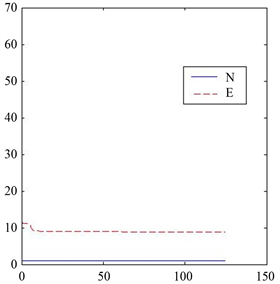Figure 1. Case I, ${\beta }_{2}=1000$ , $\delta =0.7$ , $\lambda =0.2$ , $\Omega =0.7$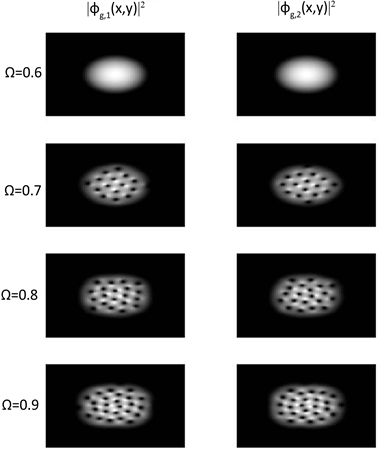Figure 2. Case I, ${\beta }_{2}=1000$ , $\delta =0.7$ , $\lambda =0.2$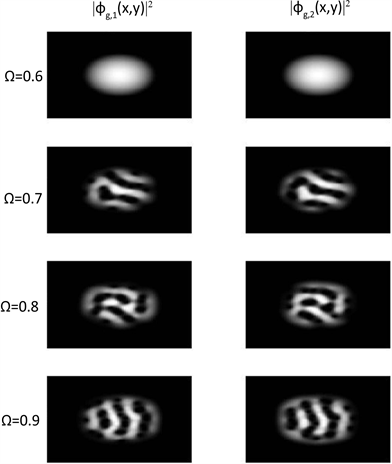Figure 3. Case I, ${\beta }_{2}=1000$ , $\delta =1.1$ , $\lambda =0.2$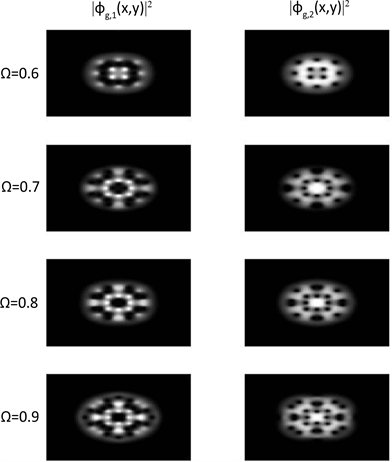Figure 4. Case II, ${\beta }_{2}=1000$ , $\lambda =0.2$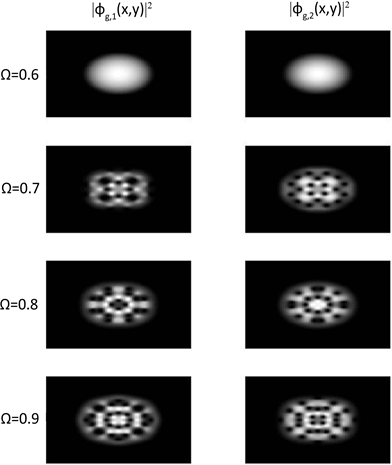Figure 5. Case III, ${\beta }_{2}=1000$ , $\lambda =0.2$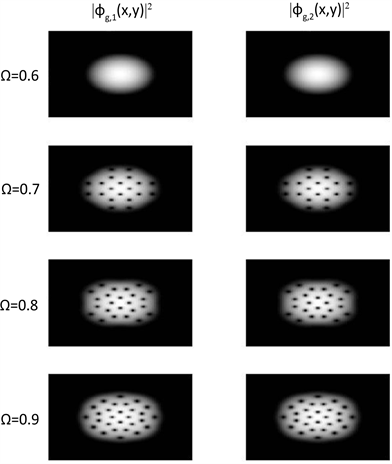Figure 6. Case III, ${\beta }_{2}=1000$ , $\lambda =2$

5. 结论

Numerical Simulations on Ground States for Rotating Two-Component Bose-Einstein Condensates[J]. 应用数学进展, 2017, 06(09): 1187-1200. http://dx.doi.org/10.12677/AAM.2017.69144

1. 1. Bao, W. and Du, Q. (2004) Computing the Ground State Solution of Bose-Einstein Condensates by a Normalized Gra-dient Flow. SIAM Journal on Scientific Computing, 25, 1674-1697.
https://doi.org/10.1137/S1064827503422956

2. 2. Kasamatsu, K., Tsubota, M. and Ueda, M. (2003) Structure of Vortex Lattices in Rotating Two-Component Bose-Einstein Condensates. Physica B: Condensed Matter, 329-333, 23-24.
https://doi.org/10.1016/S0921-4526(02)01877-X

3. 3. Miesner, H.J., Stamper-Kurn, D.M., Stenger, J., Inouye, S., Chikkatur, A.P. and Ketterle, W. (1999) Observation of Metastable States in Spinor Bose-Einstein Condensates. Physical Review Letters, 82, 2228
https://doi.org/10.1103/PhysRevLett.82.2228

4. 4. Seiringer, R. (2002) Gross-Pitaevskii Theory of the Rotating Bose Gas. Communications in Mathematical Physics, 229, 491-509.
https://doi.org/10.1007/s00220-002-0695-2

5. 5. Trippenbach, M., Góral, K., Rzazewski, K., Malomed, B. and Band, Y.B. (2000) Structure of Binary Bose-Einstein Condensates. Journal of Physics B: Atomic, Molecular and Optical Physics, 33, 4017-4031
https://doi.org/10.1088/0953-4075/33/19/314

6. 6. Wang, H. (2007) A Time-Splitting Spectral Method for Cou-pled Gross-Pitaevskii Equations with Applications to the Dynamics of Rotating Bose-Einstein Condensates. Journal of Computational and Applied Mathematics, 205, 88-104.
https://doi.org/10.1016/j.cam.2006.04.042

7. 7. Castin, Y. and Dum, R. (1999) Bose-Einstein Condensates with Vortices in Rotating Traps. The European Physical Journal D, 7, 399-412.
https://doi.org/10.1007/s100530050584

8. 8. García-Ripoll, J.J. and Pérez-García, V.M. (2002) Split Vortices in Optically Coupled Bose-Einstein Condensates. Physical Review A, 66, 021602.
https://doi.org/10.1103/PhysRevA.66.021602

9. 9. Kasamatsu, K., Tsubota, M. and Ueda, M. (2003) Vortex Phase Diagram in Rotating Two-Component Bose-Einstein Condensates. Physical Review Letters, 91, 150406.
https://doi.org/10.1103/PhysRevLett.91.150406

10. 10. Mueller, E.J. and Ho, T.-L. (2002) Two-Component Bose-Einstein Condensates with a Large Number of Vortices. Physical Review Letters, 88, 180403.
https://doi.org/10.1103/PhysRevLett.88.180403

11. 11. Schweikhard, V., Coddington, I., Engels, P., Tung, S. and Cornell, E.A. (2004) Vortex-Lattice Dynamics in Rotating Spinor Bose-Einstein Condensates. Physical Review Letters, 93, 210403.
https://doi.org/10.1103/PhysRevLett.93.210403

12. 12. Bao, W. (2004) Ground States and Dynamics of Mul-ti-Component Bose-Einstein Condensates. SIAM Multiscale Modeling & Simulation, 2, 210-236.
https://doi.org/10.1137/030600209

13. 13. Chang, S.M., Lin, W.W. and Shieh, S.F. (2005) Gauss-Seidel-Type Methods for Energy States of Multi-Component Bose-Einstein Condensates. Journal of Computational Physics, 202, 367-390.
https://doi.org/10.1016/j.jcp.2004.07.012

14. 14. Pu, H. and Bigelow, N.P. (1998) Properties of Two-Species Bose Condensates. Physical Review Letters, 80, 1130.
https://doi.org/10.1103/PhysRevLett.80.1130

15. 15. Riboli, F. and Modugno, M. (2002) Topology of the Ground State of Two Interacting Bose-Einstein Condensates. Physical Review A, 65, 063614.
https://doi.org/10.1103/PhysRevA.65.063614

16. 16. Esry, B.D. and Greene, C.H. (1999) Spontaneous Spatial Symmetry Breaking in Two-Component Bose-Einstein Condensates. Physical Review A, 59, 1457-1460.
https://doi.org/10.1103/PhysRevA.59.1457

17. 17. Chui, S.T., Ryzhov, V.N. and Tareyeva, E.E. (2001) Vortex States in Binary Mixture of Bose-Einstein Condensates. Physical Review A, 63, 023605.
https://doi.org/10.1103/PhysRevA.63.023605

18. 18. García-Ripoll, J.J. and Pérez-García, V.M. (2000) Stable and Unstable Vortices in Multicomponent Bose-Einstein Condensates. Physical Review Letters, 81, 4264.
https://doi.org/10.1103/PhysRevLett.84.4264

19. 19. Ho, T.L. and Shenoy, V.B. (1996) Binary Mixtures of Bose Condensates of Alkali Atoms. Physical Review Letters, 77, 3276.
https://doi.org/10.1103/PhysRevLett.77.3276

20. 20. Vekslerchik, V. and Pérez-García, V.M. (2003) Exact Solution of the Two-Mode Model of Multicomponent Bose- Einstein Condensates. Discrete & Continuous Dynamical Sys-tems—B, 3, 179-192.
https://doi.org/10.3934/dcdsb.2003.3.179

21. 21. Battye, R.A., Cooper, N.R. and Sutcliffe, P.M. (2002) Stable Skyrmions in Two-Component Bose-Einstein Condensates. Physical Review Letters, 88, 080401.
https://doi.org/10.1103/PhysRevLett.88.080401

22. 22. Wang, H. (2009) Numerical Simulations on Stationary States for Rotating Two-Component Bose-Einstein Condensates. Journal of Scientific Computing, 38, 149-163.
https://doi.org/10.1007/s10915-008-9225-5

23. 23. Zhang, Y., Bao, W. and Li, H. (2007) Dynamics of Rotating Two-Component Bose-Einstein Condensates and Its Efficient Computation. Physica D, 234, 49-69.
https://doi.org/10.1016/j.physd.2007.06.026

24. 24. Bao, W., Wang, H.Q. and Markowich, P.A. (2005) Ground State, Symmetric and Central Vortex State in Rotating Bose-Einstein Condensate. Communications in Mathematical Sciences, 3, 57-88.
https://doi.org/10.4310/CMS.2005.v3.n1.a5

25. 25. Hall, D.S., Matthews, M.R., Ensher, J.R., Wieman, C.E. and Cornell, E.A. (1998) Dynamics of Component Separation in a Binary Mixture of Bose-Einstein Condensates. Physical Review Letters, 81, 1539-1542.
https://doi.org/10.1103/PhysRevLett.81.1539